Скачать презентацию TASC Topology Adaptive Spatial Clustering for Sensor Networks

• Количество слайдов: 35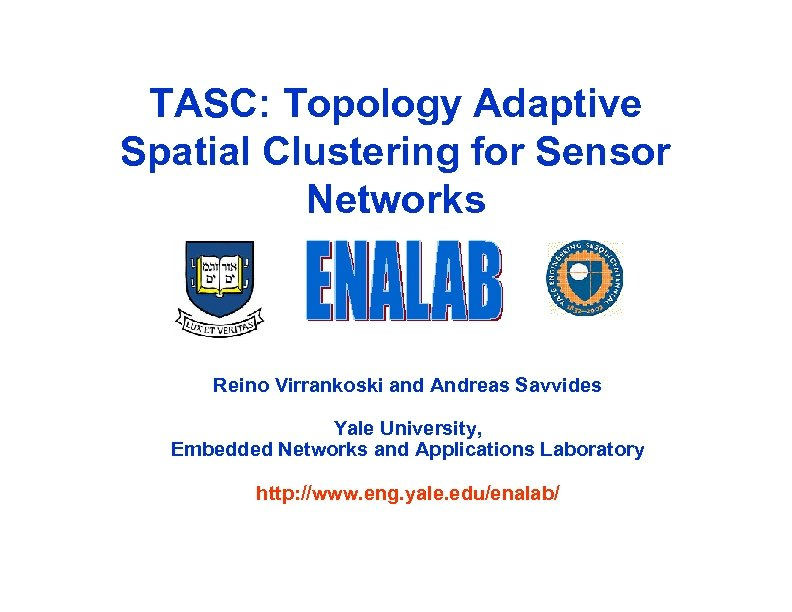TASC: Topology Adaptive Spatial Clustering for Sensor Networks Reino Virrankoski and Andreas Savvides Yale University, Embedded Networks and Applications Laboratory http: //www. eng. yale. edu/enalab/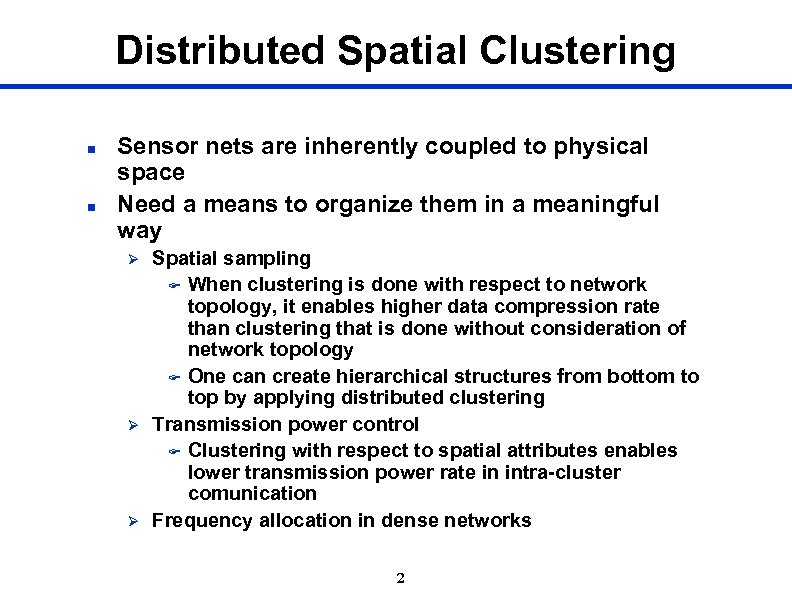Distributed Spatial Clustering n n Sensor nets are inherently coupled to physical space Need a means to organize them in a meaningful way Ø Ø Ø Spatial sampling F When clustering is done with respect to network topology, it enables higher data compression rate than clustering that is done without consideration of network topology F One can create hierarchical structures from bottom to top by applying distributed clustering Transmission power control F Clustering with respect to spatial attributes enables lower transmission power rate in intra-cluster comunication Frequency allocation in dense networks 2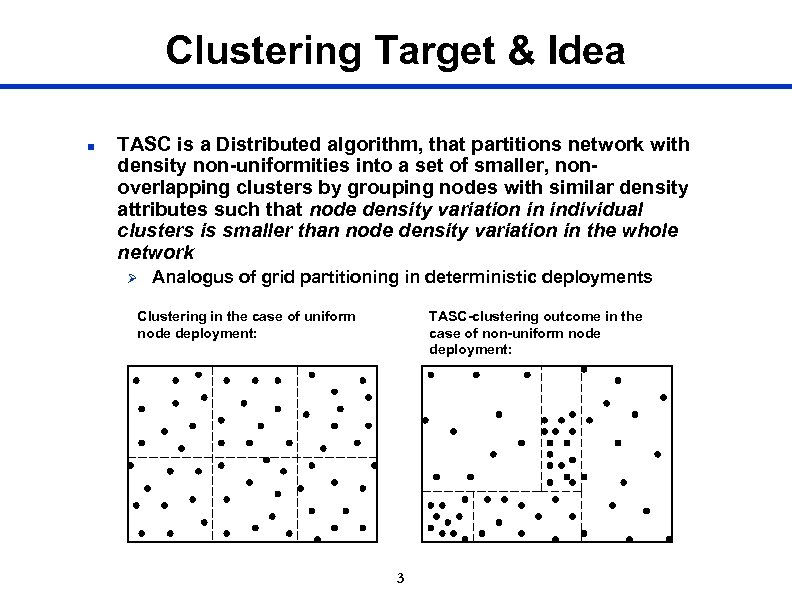Clustering Target & Idea n TASC is a Distributed algorithm, that partitions network with density non-uniformities into a set of smaller, nonoverlapping clusters by grouping nodes with similar density attributes such that node density variation in individual clusters is smaller than node density variation in the whole network Ø Analogus of grid partitioning in deterministic deployments TASC-clustering outcome in the case of non-uniform node deployment: Clustering in the case of uniform node deployment: 3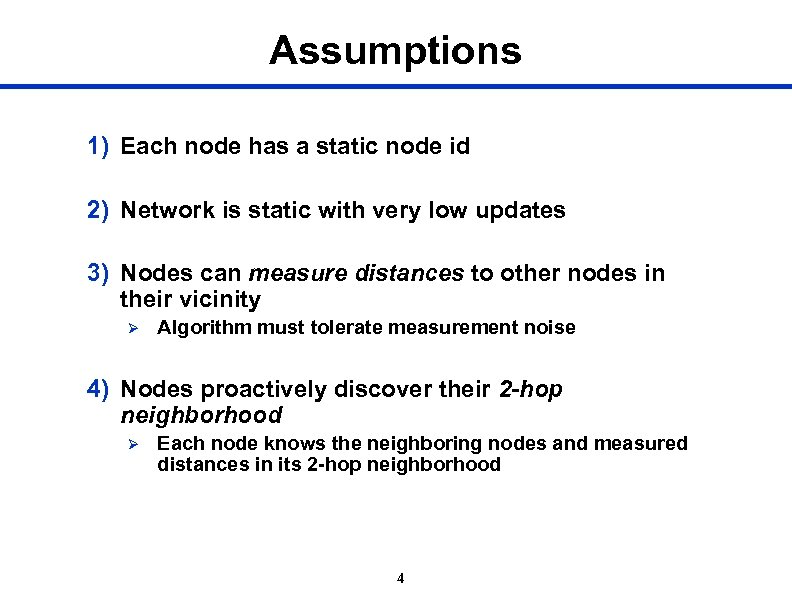Assumptions 1) Each node has a static node id 2) Network is static with very low updates 3) Nodes can measure distances to other nodes in their vicinity Ø Algorithm must tolerate measurement noise 4) Nodes proactively discover their 2 -hop neighborhood Ø Each node knows the neighboring nodes and measured distances in its 2 -hop neighborhood 4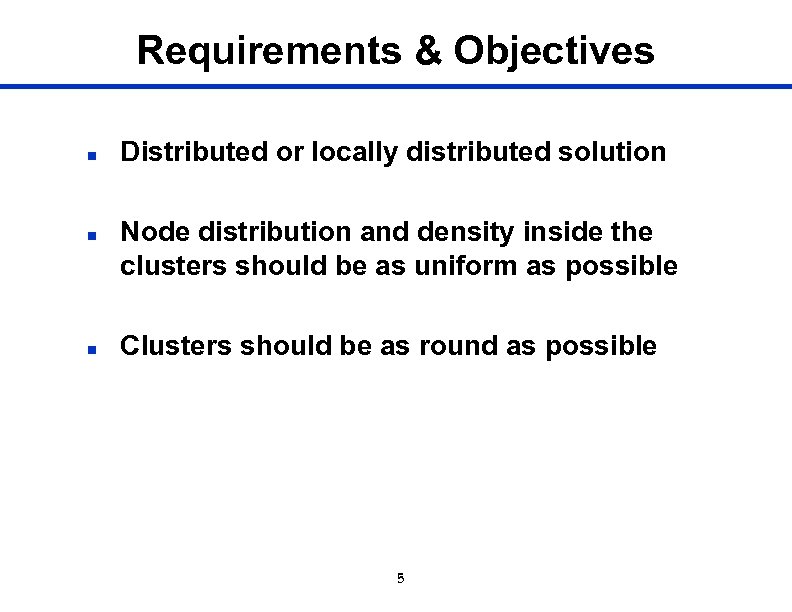Requirements & Objectives n n n Distributed or locally distributed solution Node distribution and density inside the clusters should be as uniform as possible Clusters should be as round as possible 5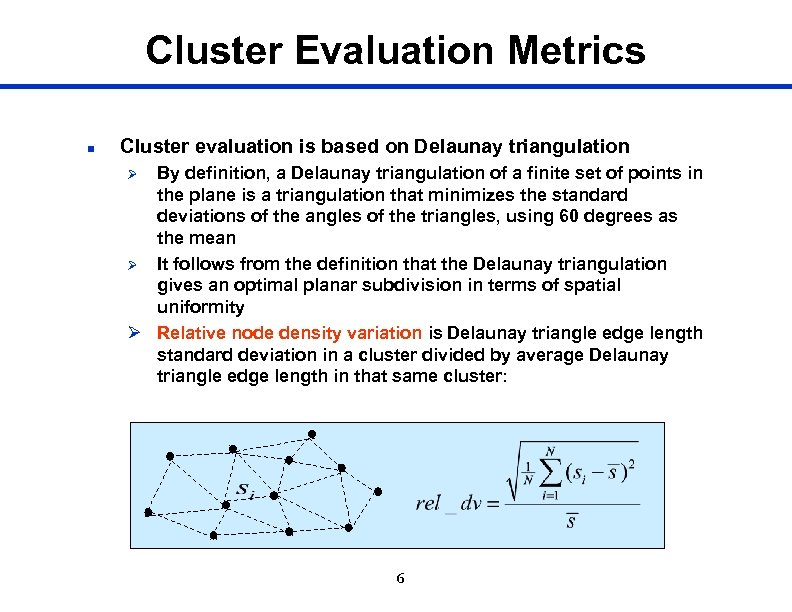Cluster Evaluation Metrics n Cluster evaluation is based on Delaunay triangulation By definition, a Delaunay triangulation of a finite set of points in the plane is a triangulation that minimizes the standard deviations of the angles of the triangles, using 60 degrees as the mean Ø It follows from the definition that the Delaunay triangulation gives an optimal planar subdivision in terms of spatial uniformity Ø Relative node density variation is Delaunay triangle edge length standard deviation in a cluster divided by average Delaunay triangle edge length in that same cluster: Ø 6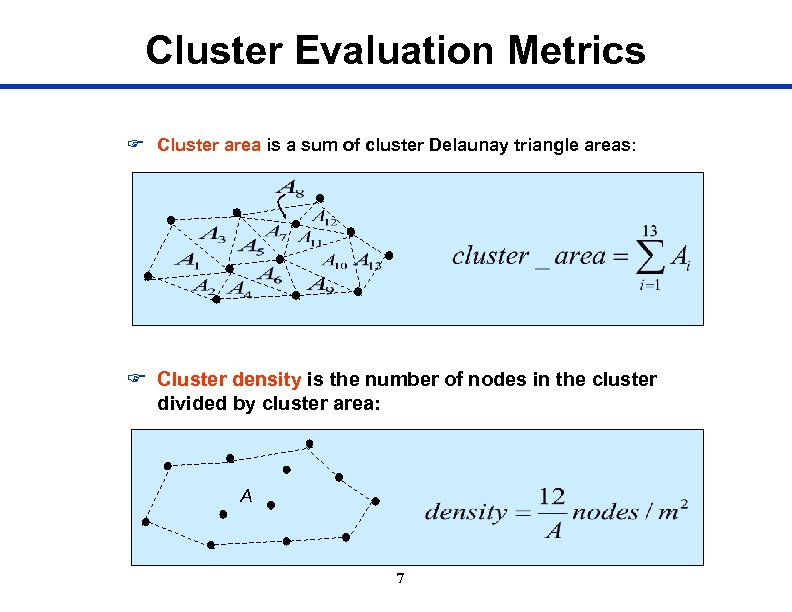Cluster Evaluation Metrics F Cluster area is a sum of cluster Delaunay triangle areas: F Cluster density is the number of nodes in the cluster divided by cluster area: A 7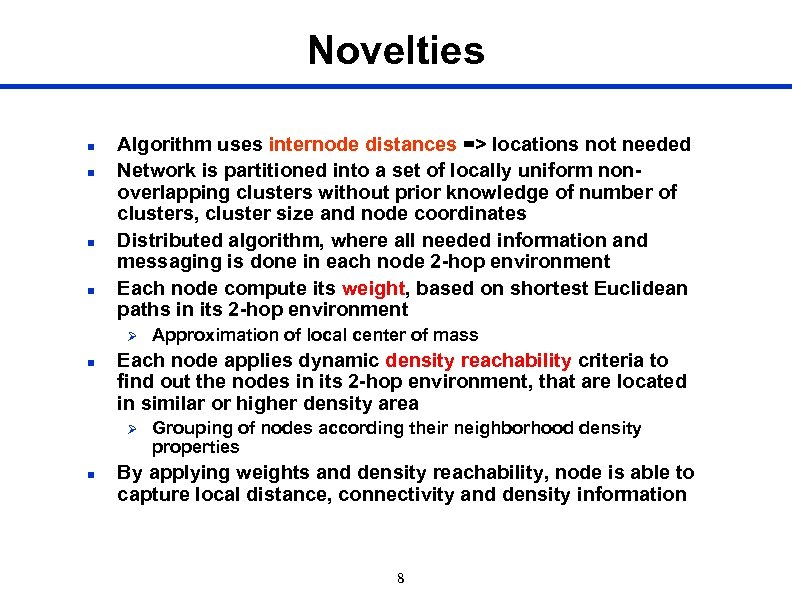Novelties n n Algorithm uses internode distances => locations not needed Network is partitioned into a set of locally uniform nonoverlapping clusters without prior knowledge of number of clusters, cluster size and node coordinates Distributed algorithm, where all needed information and messaging is done in each node 2 -hop environment Each node compute its weight, based on shortest Euclidean paths in its 2 -hop environment Ø n Each node applies dynamic density reachability criteria to find out the nodes in its 2 -hop environment, that are located in similar or higher density area Ø n Approximation of local center of mass Grouping of nodes according their neighborhood density properties By applying weights and density reachability, node is able to capture local distance, connectivity and density information 8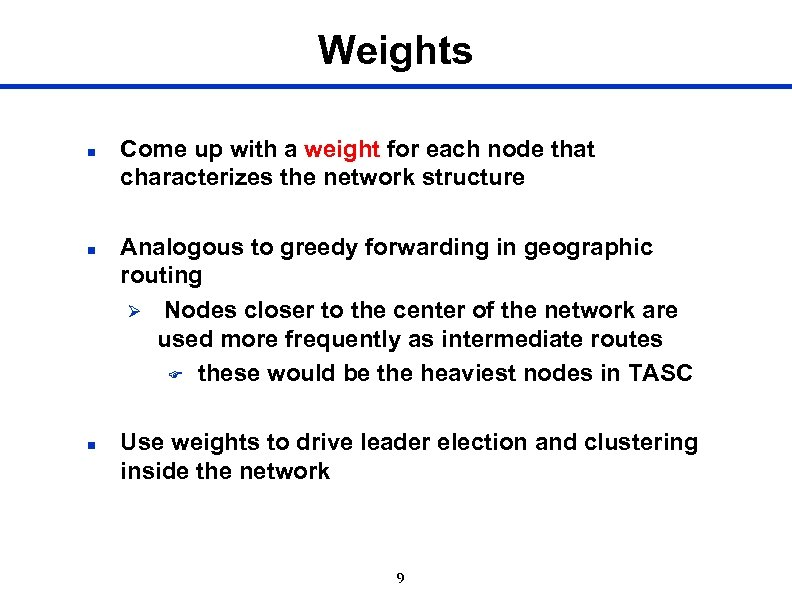Weights n n n Come up with a weight for each node that characterizes the network structure Analogous to greedy forwarding in geographic routing Ø Nodes closer to the center of the network are used more frequently as intermediate routes F these would be the heaviest nodes in TASC Use weights to drive leader election and clustering inside the network 9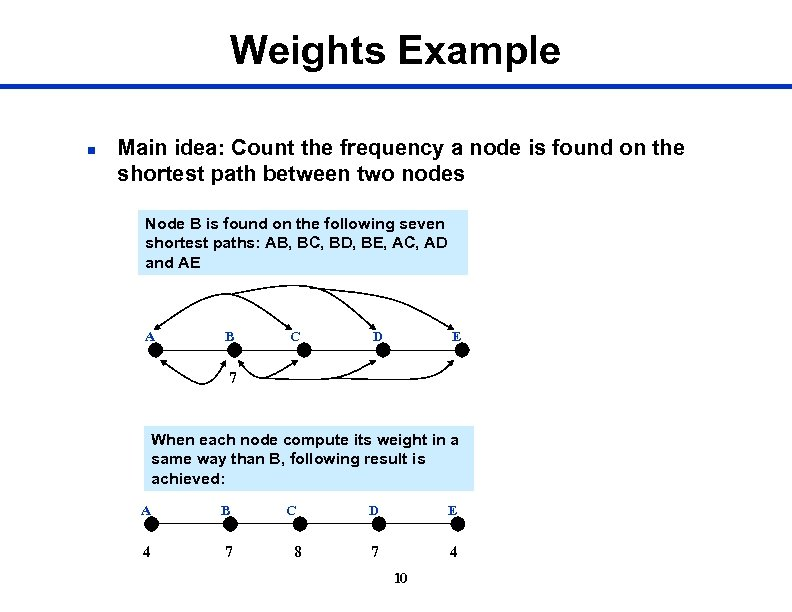Weights Example n Main idea: Count the frequency a node is found on the shortest path between two nodes Node B is found on the following seven shortest paths: AB, BC, BD, BE, AC, AD and AE A B C D E 7 When each node compute its weight in a same way than B, following result is achieved: A B 4 7 C 8 D E 7 4 10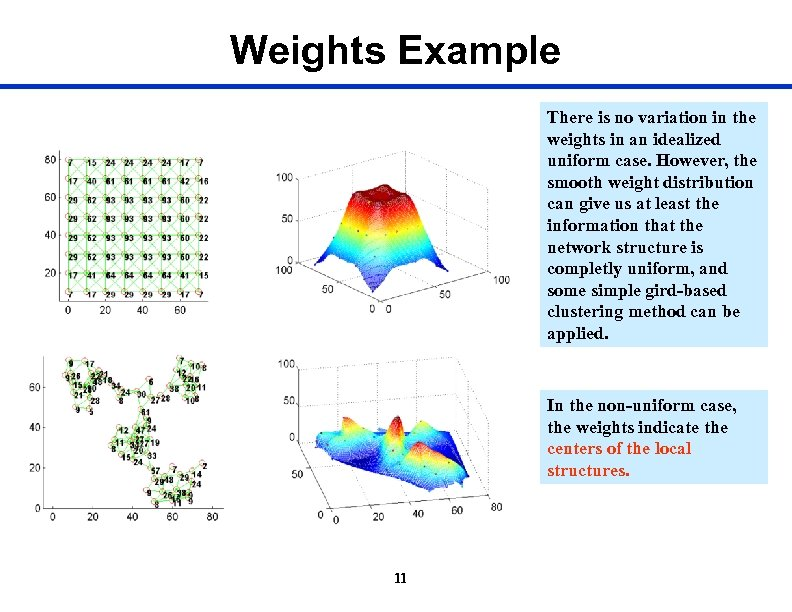Weights Example There is no variation in the weights in an idealized uniform case. However, the smooth weight distribution can give us at least the information that the network structure is completly uniform, and some simple gird-based clustering method can be applied. In the non-uniform case, the weights indicate the centers of the local structures. 11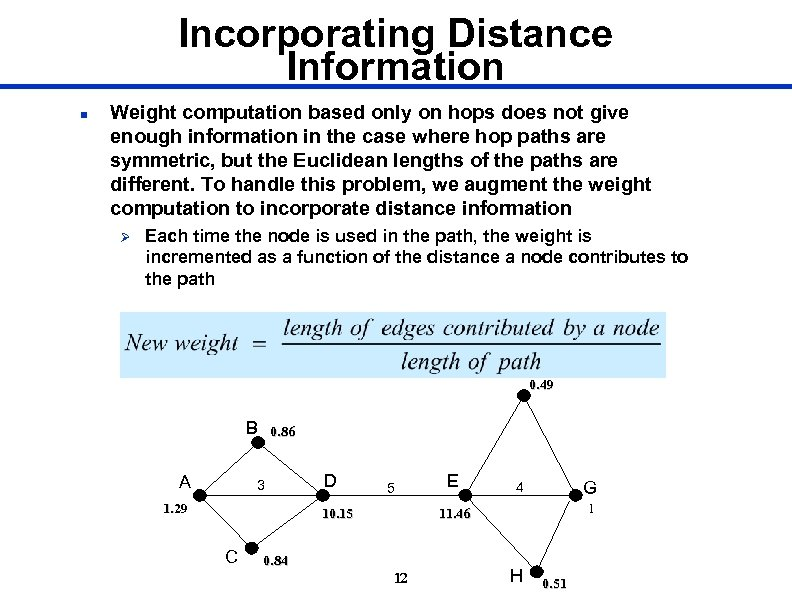Incorporating Distance Information n Weight computation based only on hops does not give enough information in the case where hop paths are symmetric, but the Euclidean lengths of the paths are different. To handle this problem, we augment the weight computation to incorporate distance information Ø Each time the node is used in the path, the weight is incremented as a function of the distance a node contributes to the path 0. 49 B A 0. 86 3 1. 29 D 5 10. 15 C E G 4 1 11. 46 0. 84 12 H 0. 51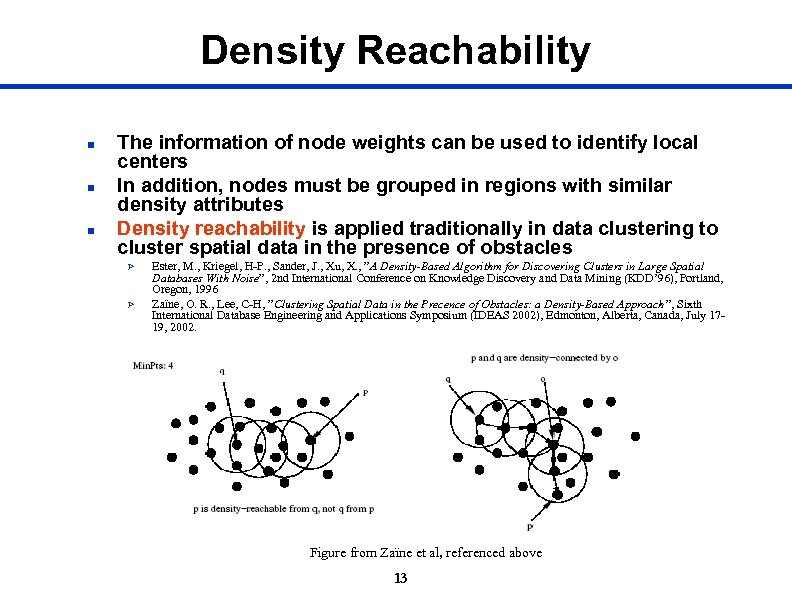Density Reachability n n n The information of node weights can be used to identify local centers In addition, nodes must be grouped in regions with similar density attributes Density reachability is applied traditionally in data clustering to cluster spatial data in the presence of obstacles Ø Ø Ester, M. , Kriegel, H-P. , Sander, J. , Xu, X. , ”A Density-Based Algorithm for Discovering Clusters in Large Spatial Databases With Noise”, 2 nd International Conference on Knowledge Discovery and Data Mining (KDD’ 96), Portland, Oregon, 1996 Zaïne, O. R. , Lee, C-H, ”Clustering Spatial Data in the Precence of Obstacles: a Density-Based Approach ”, Sixth International Database Engineering and Applications Symposium (IDEAS 2002), Edmonton, Alberta, Canada, July 1719, 2002. Figure from Zaïne et al, referenced above 13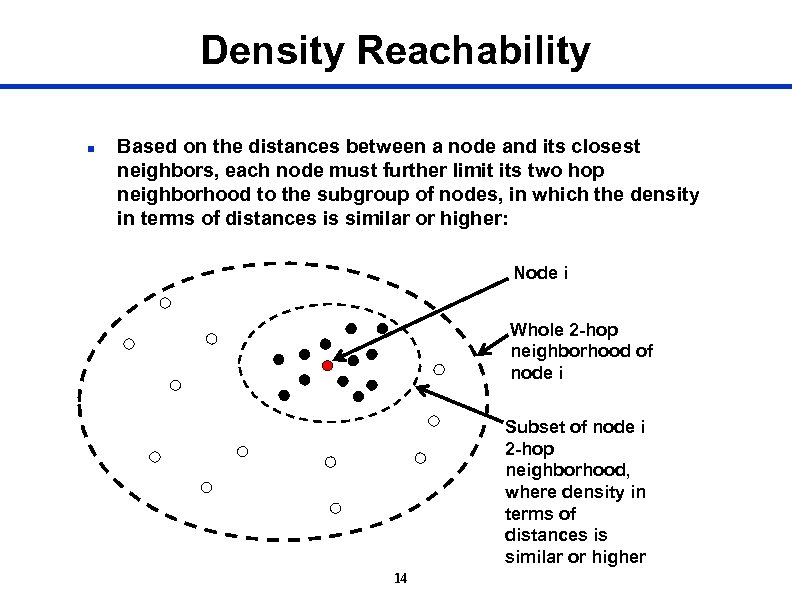Density Reachability n Based on the distances between a node and its closest neighbors, each node must further limit its two hop neighborhood to the subgroup of nodes, in which the density in terms of distances is similar or higher: Node i Whole 2 -hop neighborhood of node i Subset of node i 2 -hop neighborhood, where density in terms of distances is similar or higher 14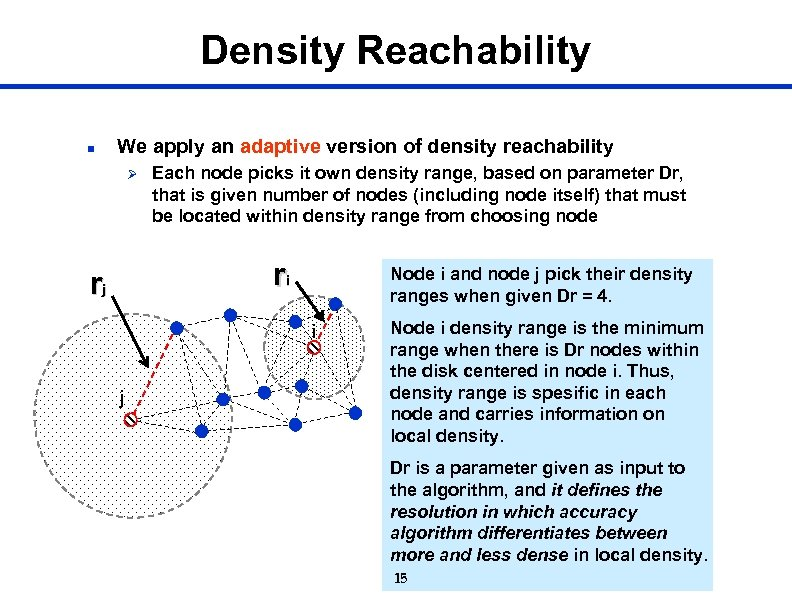Density Reachability n We apply an adaptive version of density reachability Ø Each node picks it own density range, based on parameter Dr, that is given number of nodes (including node itself) that must be located within density range from choosing node ri rj Node i and node j pick their density ranges when given Dr = 4. i j Node i density range is the minimum range when there is Dr nodes within the disk centered in node i. Thus, density range is spesific in each node and carries information on local density. Dr is a parameter given as input to the algorithm, and it defines the resolution in which accuracy algorithm differentiates between more and less dense in local density. 15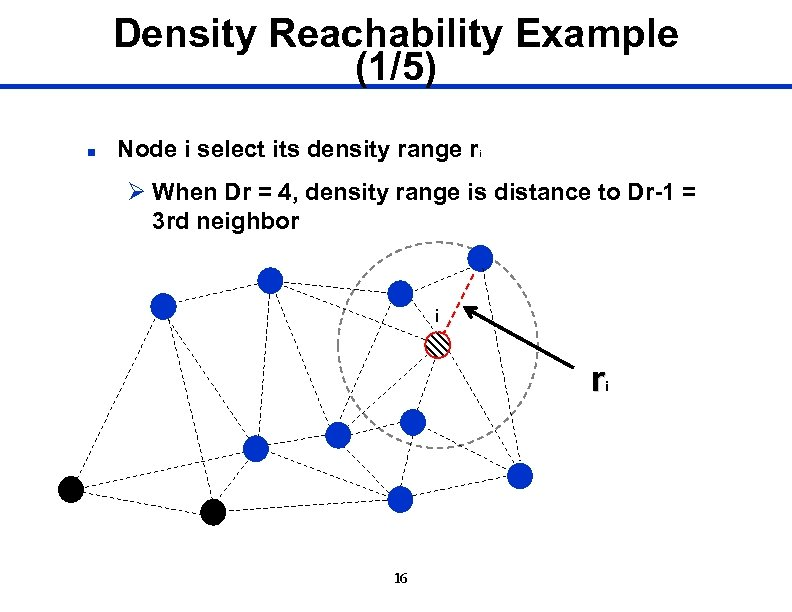Density Reachability Example (1/5) n Node i select its density range ri Ø When Dr = 4, density range is distance to Dr-1 = 3 rd neighbor i 16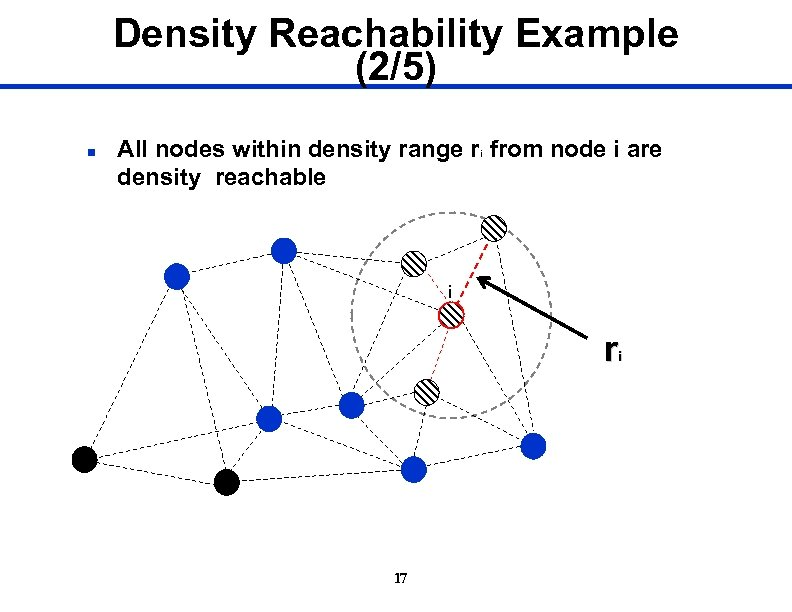Density Reachability Example (2/5) n All nodes within density range ri from node i are density reachable i r i 17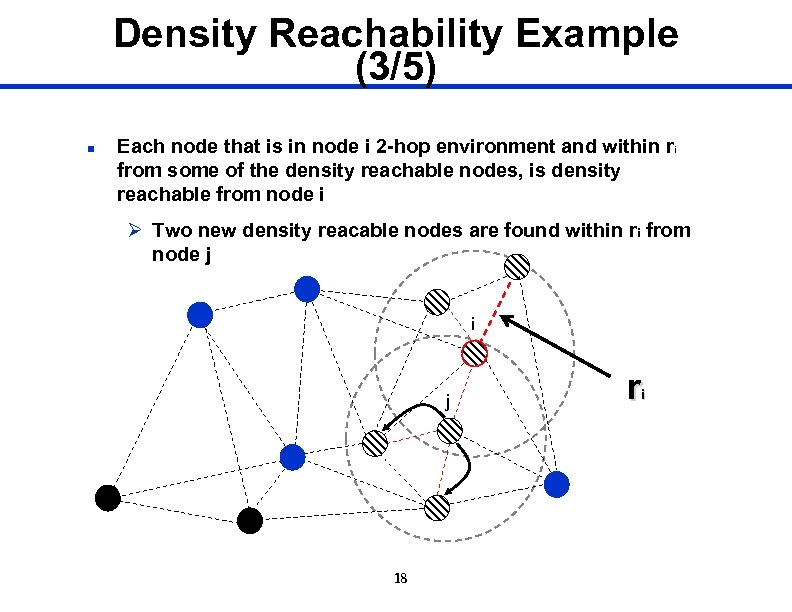Density Reachability Example (3/5) n Each node that is in node i 2 -hop environment and within r i from some of the density reachable nodes, is density reachable from node i Ø Two new density reacable nodes are found within ri from node j i j 18 r i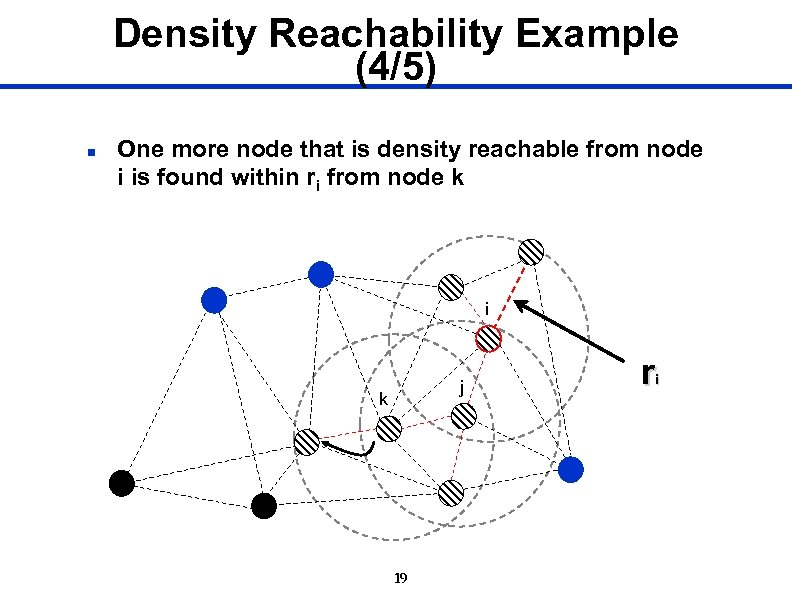Density Reachability Example (4/5) n One more node that is density reachable from node i is found within ri from node k i j k 19 r i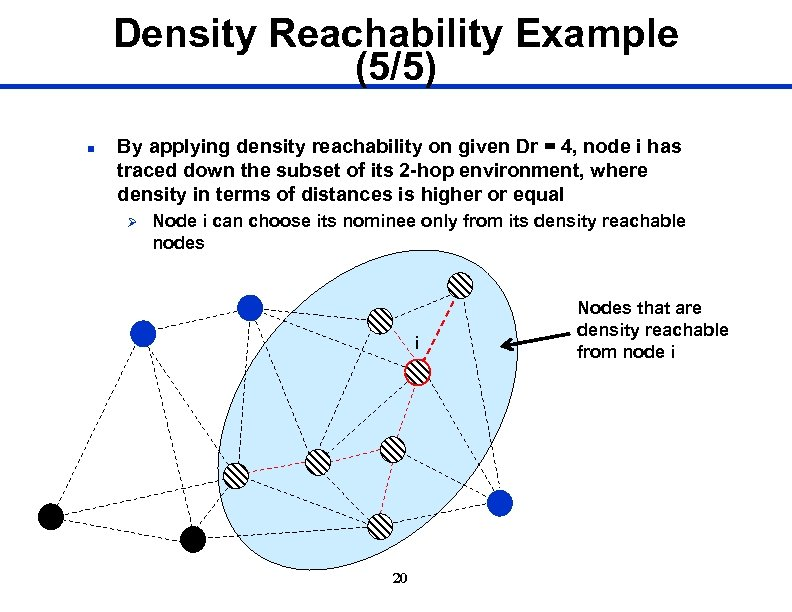Density Reachability Example (5/5) n By applying density reachability on given Dr = 4, node i has traced down the subset of its 2 -hop environment, where density in terms of distances is higher or equal Ø Node i can choose its nominee only from its density reachable nodes i 20 Nodes that are density reachable from node i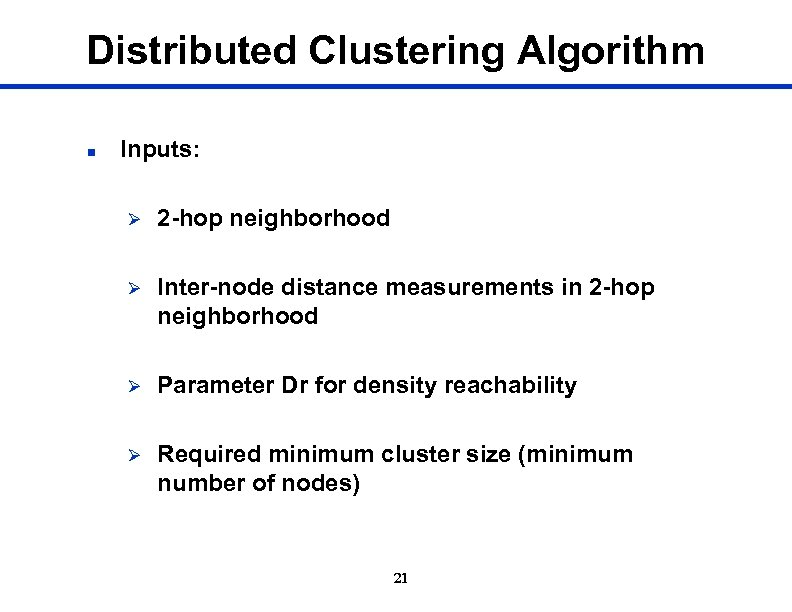Distributed Clustering Algorithm n Inputs: Ø 2 -hop neighborhood Ø Inter-node distance measurements in 2 -hop neighborhood Ø Parameter Dr for density reachability Ø Required minimum cluster size (minimum number of nodes) 21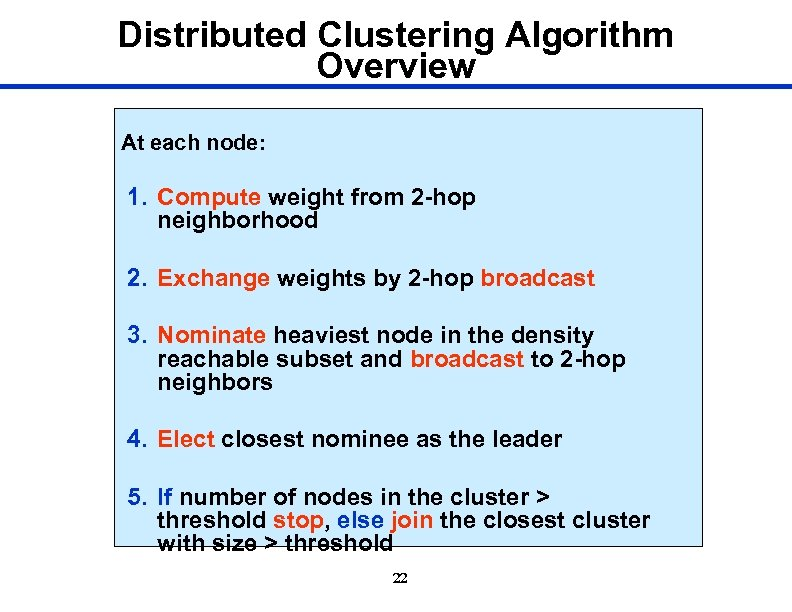Distributed Clustering Algorithm Overview At each node: 1. Compute weight from 2 -hop neighborhood 2. Exchange weights by 2 -hop broadcast 3. Nominate heaviest node in the density reachable subset and broadcast to 2 -hop neighbors 4. Elect closest nominee as the leader 5. If number of nodes in the cluster > threshold stop, else join the closest cluster with size > threshold 22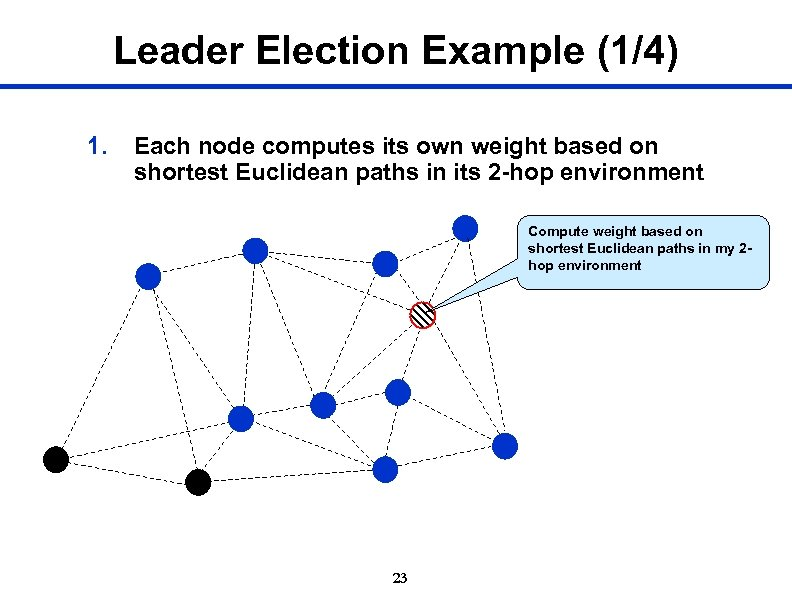Leader Election Example (1/4) 1. Each node computes its own weight based on shortest Euclidean paths in its 2 -hop environment Compute weight based on shortest Euclidean paths in my 2 hop environment 23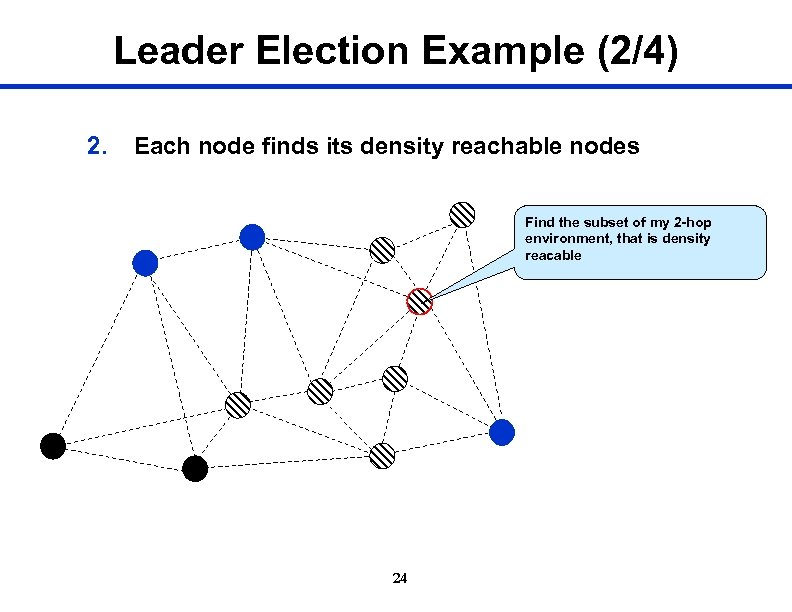Leader Election Example (2/4) 2. Each node finds its density reachable nodes Find the subset of my 2 -hop environment, that is density reacable 24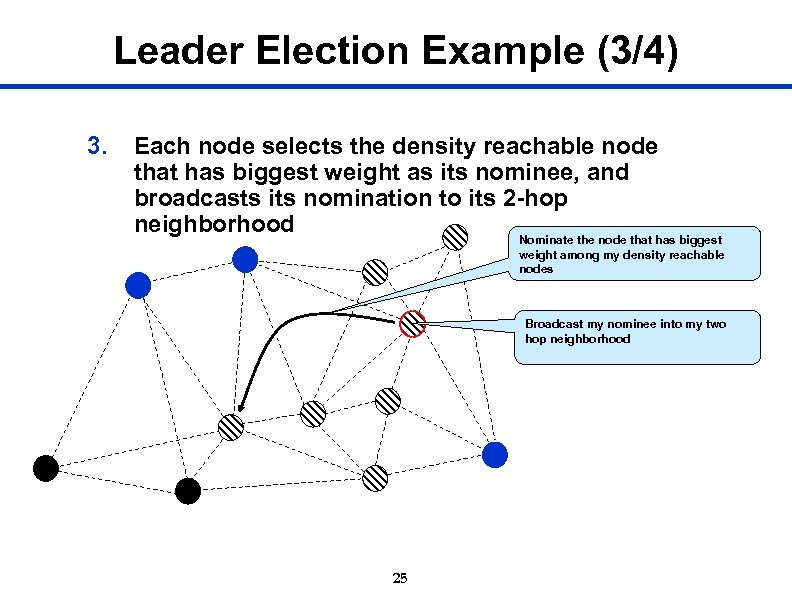Leader Election Example (3/4) 3. Each node selects the density reachable node that has biggest weight as its nominee, and broadcasts its nomination to its 2 -hop neighborhood Nominate the node that has biggest weight among my density reachable nodes Broadcast my nominee into my two hop neighborhood 25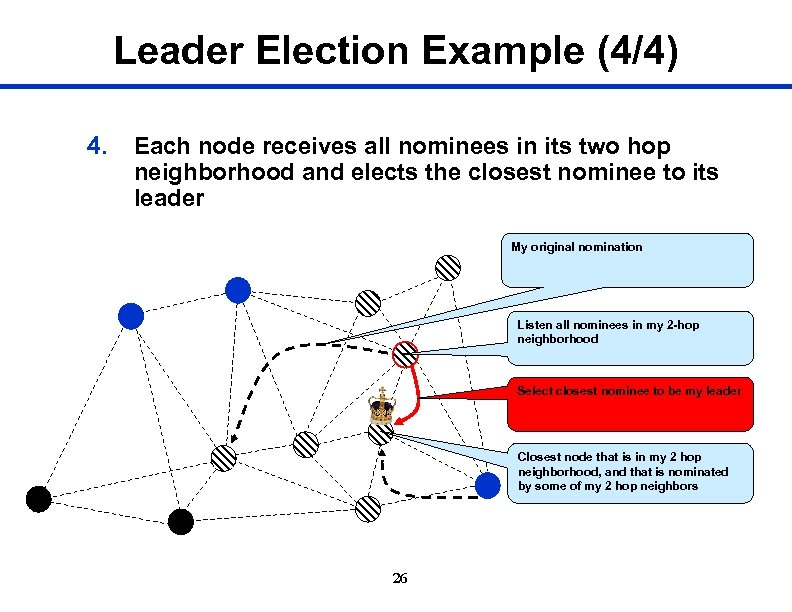Leader Election Example (4/4) 4. Each node receives all nominees in its two hop neighborhood and elects the closest nominee to its leader My original nomination Listen all nominees in my 2 -hop neighborhood Select closest nominee to be my leader Closest node that is in my 2 hop neighborhood, and that is nominated by some of my 2 hop neighbors 26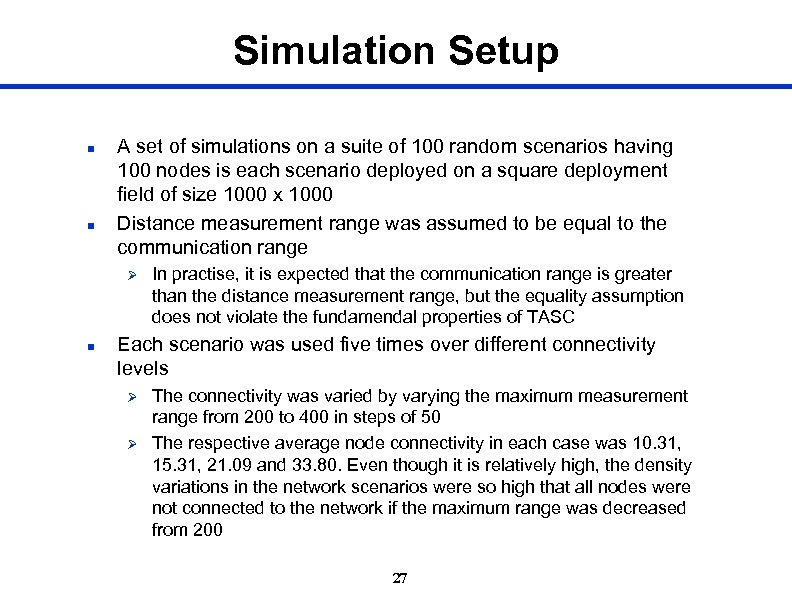Simulation Setup n n A set of simulations on a suite of 100 random scenarios having 100 nodes is each scenario deployed on a square deployment field of size 1000 x 1000 Distance measurement range was assumed to be equal to the communication range Ø n In practise, it is expected that the communication range is greater than the distance measurement range, but the equality assumption does not violate the fundamendal properties of TASC Each scenario was used five times over different connectivity levels Ø Ø The connectivity was varied by varying the maximum measurement range from 200 to 400 in steps of 50 The respective average node connectivity in each case was 10. 31, 15. 31, 21. 09 and 33. 80. Even though it is relatively high, the density variations in the network scenarios were so high that all nodes were not connected to the network if the maximum range was decreased from 200 27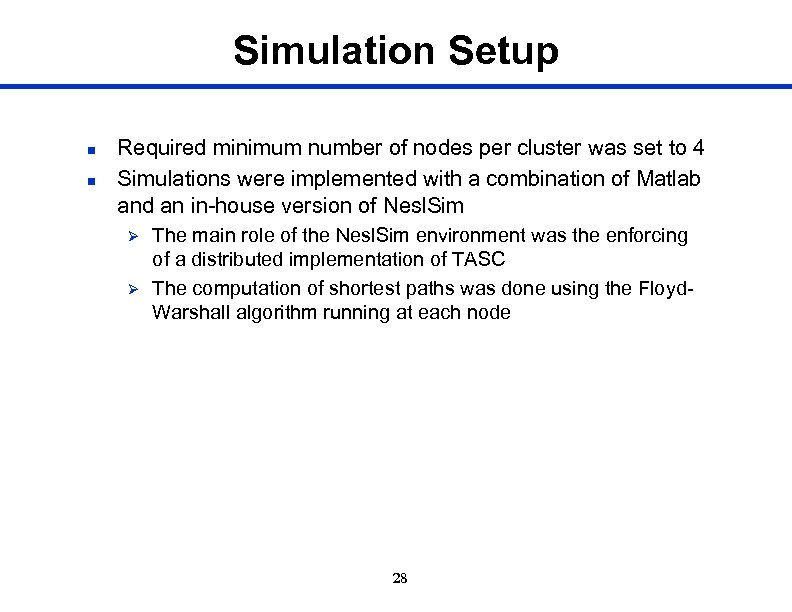Simulation Setup n n Required minimum number of nodes per cluster was set to 4 Simulations were implemented with a combination of Matlab and an in-house version of Nesl. Sim Ø Ø The main role of the Nesl. Sim environment was the enforcing of a distributed implementation of TASC The computation of shortest paths was done using the Floyd. Warshall algorithm running at each node 28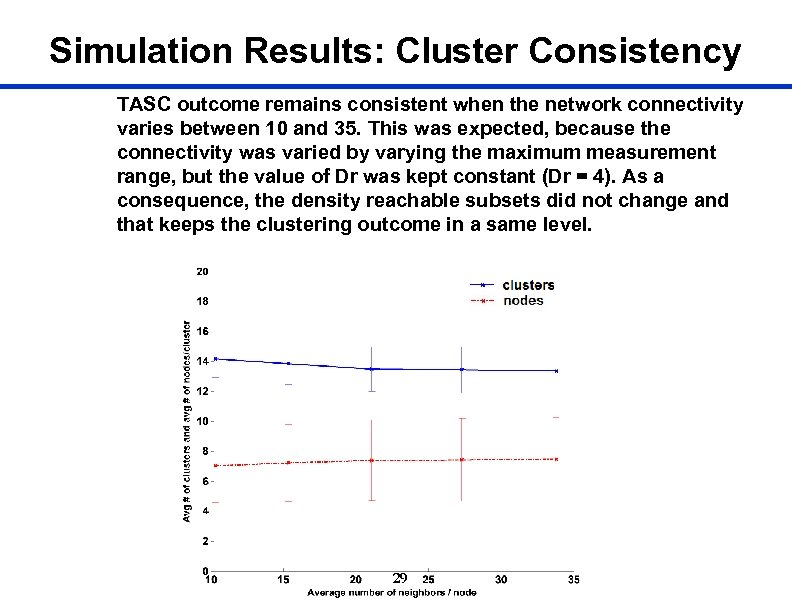Simulation Results: Cluster Consistency TASC outcome remains consistent when the network connectivity varies between 10 and 35. This was expected, because the connectivity was varied by varying the maximum measurement range, but the value of Dr was kept constant (Dr = 4). As a consequence, the density reachable subsets did not change and that keeps the clustering outcome in a same level. 29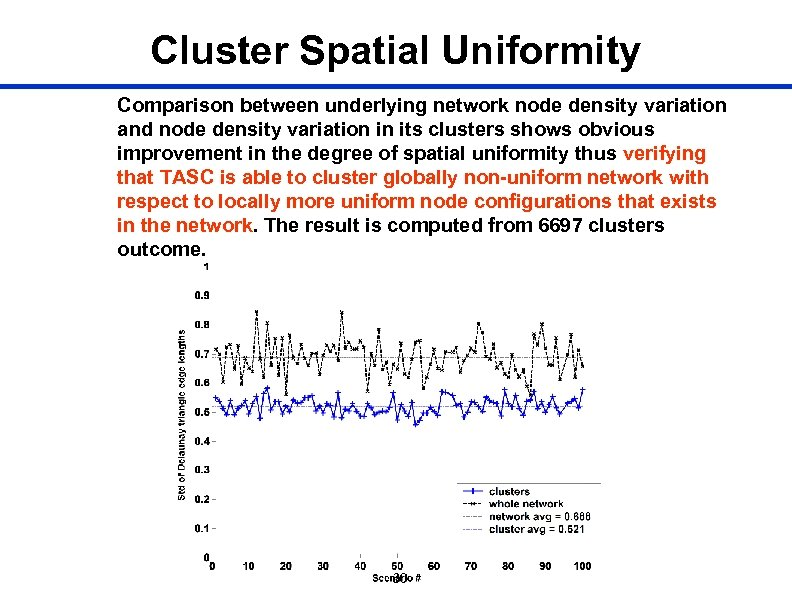Cluster Spatial Uniformity Comparison between underlying network node density variation and node density variation in its clusters shows obvious improvement in the degree of spatial uniformity thus verifying that TASC is able to cluster globally non-uniform network with respect to locally more uniform node configurations that exists in the network. The result is computed from 6697 clusters outcome. 30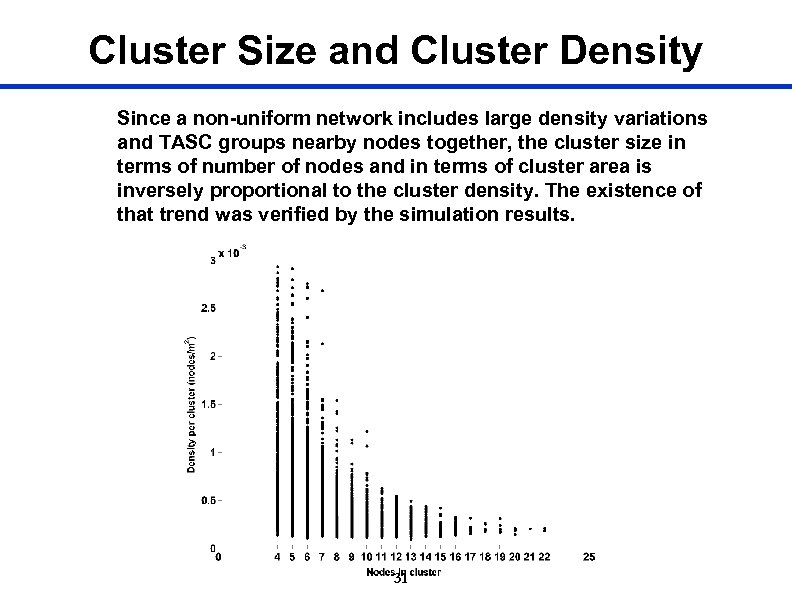Cluster Size and Cluster Density Since a non-uniform network includes large density variations and TASC groups nearby nodes together, the cluster size in terms of number of nodes and in terms of cluster area is inversely proportional to the cluster density. The existence of that trend was verified by the simulation results. 31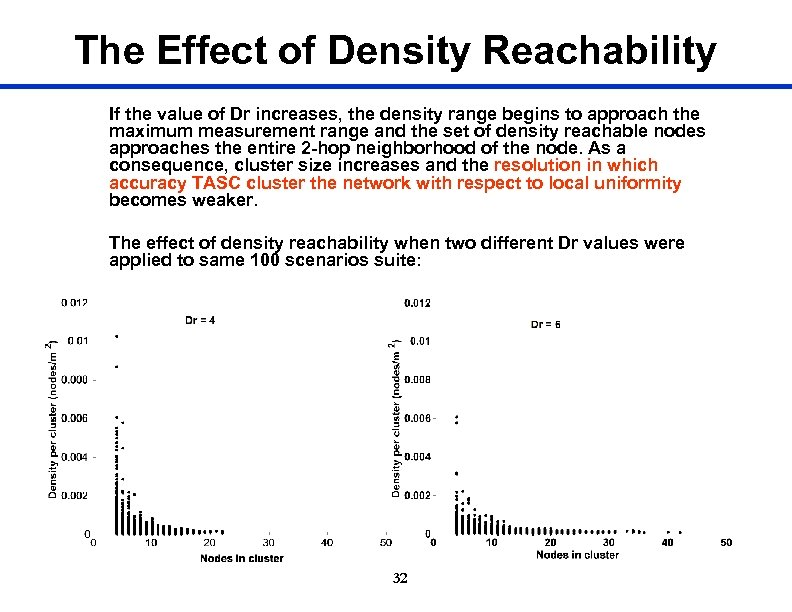The Effect of Density Reachability If the value of Dr increases, the density range begins to approach the maximum measurement range and the set of density reachable nodes approaches the entire 2 -hop neighborhood of the node. As a consequence, cluster size increases and the resolution in which accuracy TASC cluster the network with respect to local uniformity becomes weaker. The effect of density reachability when two different Dr values were applied to same 100 scenarios suite: 32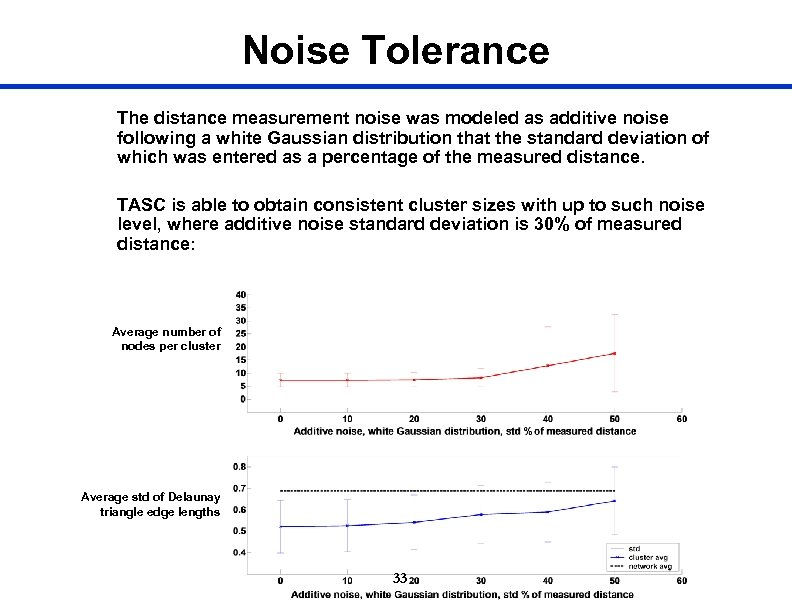Noise Tolerance The distance measurement noise was modeled as additive noise following a white Gaussian distribution that the standard deviation of which was entered as a percentage of the measured distance. TASC is able to obtain consistent cluster sizes with up to such noise level, where additive noise standard deviation is 30% of measured distance: Average number of nodes per cluster Average std of Delaunay triangle edge lengths 33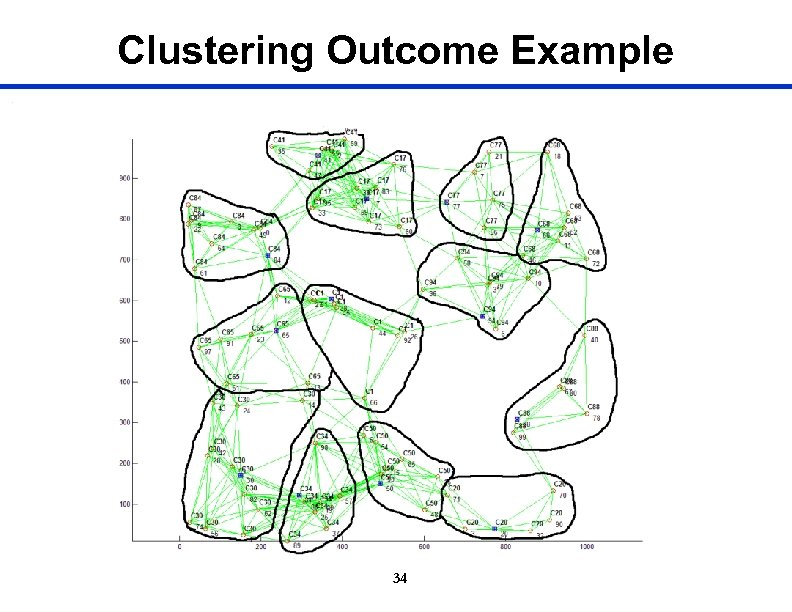Clustering Outcome Example 34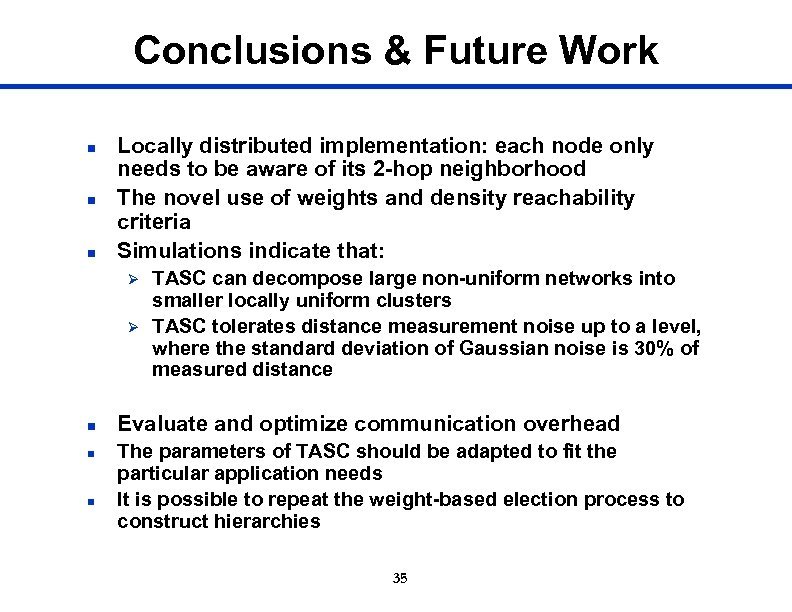Conclusions & Future Work n n n Locally distributed implementation: each node only needs to be aware of its 2 -hop neighborhood The novel use of weights and density reachability criteria Simulations indicate that: Ø Ø n n n TASC can decompose large non-uniform networks into smaller locally uniform clusters TASC tolerates distance measurement noise up to a level, where the standard deviation of Gaussian noise is 30% of measured distance Evaluate and optimize communication overhead The parameters of TASC should be adapted to fit the particular application needs It is possible to repeat the weight-based election process to construct hierarchies 35